### 奥迪(进口) 奥迪A1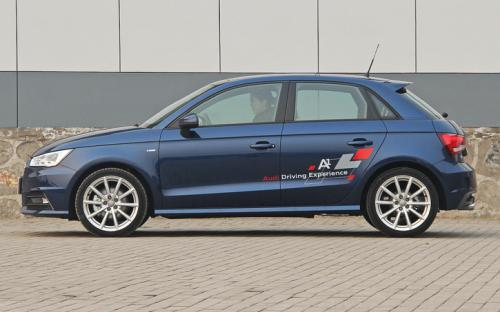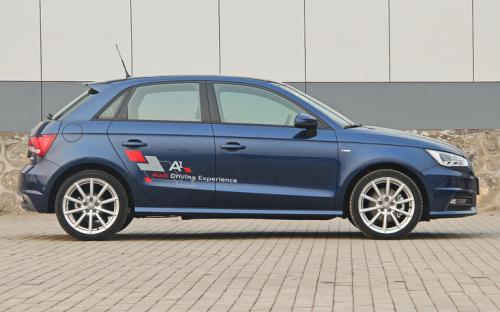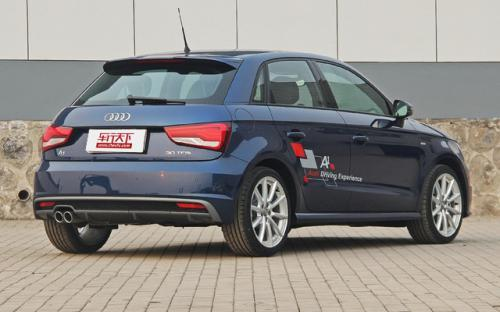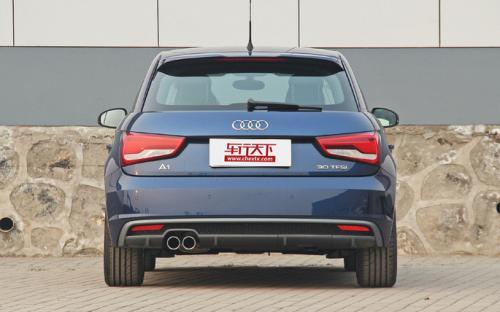10 种颜色可选

SOHO棕冰川白花剑银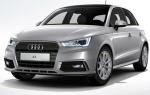米萨诺红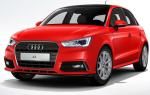米索斯黑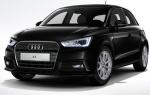纳诺灰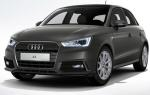斯科巴蓝乌托邦蓝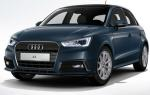旋风黑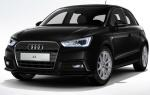真丝白2016款最低售价：20.48 万元起

3987(mm)1746(mm)1422(mm)##### 配置亮点：
• 胎压监测装置

• ISOFIX儿童座椅接口

• 车身稳定控制(ESC/ESP/DSC等)

• 电动天窗

• 定速巡航

• 后倒车雷达

• 真皮座椅

• GPS导航系统

• 氙气大灯

• 后视镜加热

• 提交
2016款 30 TFSI Sportback S Line运动版 (235张)

奥迪(进口) 奥迪A1 绕车实拍• 奥迪(进口) 奥迪A1 在售车型

排量 车型 厂商指导价 本地最低报价 购车工具
1.4L
23.48万
1.4T
20.48万

奥迪(进口) 奥迪A1 经销商

查看更多 >>

### 奥迪(进口) 奥迪A1 动力加速

奥迪A1 0-100公里加速时间分布在 8.9-8.9秒 属于 运动级

动力级别 加速时间 车型
运动级(1款)
8.9s
30 TFSI Sportback S Line运动版30 TFSI Sportback Design风尚版

奥迪(进口) 奥迪A1 视频

奥迪(进口) 奥迪A1 新闻资讯

# 新款奥迪A1 Sportback上市 售价20.48万起

进口新车 超过8292次关注

日前，我们从奥迪官方获悉，2016款奥迪A1Sportback于1月4日正式上市，新车共推出Design风尚版和SLine运动版两款车型，售价分别为20.48万元和23.48万元。

# 或2016年亮相 奥迪新一代A1信息曝光

进口新车 超过4307次关注

日前，国外媒体报道，在奥迪追加研发投入的报告中显示，奥迪将会在2016年推出全新一代A1。

# 摩纳哥试奥迪新款A1 1.0TFSI 3缸也给力

评测 超过5793次关注

摩纳哥是个土豪扎堆儿的地方，满大街跑豪车就不说了，港湾里的游艇都停满了。这地方仅次于梵蒂冈，是世界上第二小的国家，又是人口最稠密的国家。各种人啊车啊汇聚...

# 造型小改 奥迪新款A1/A1 Sportback官图

进口新车 超过4990次关注

日前，奥迪官方正式公布了新款A1/A1Sportback两款车型的官图（文中统一称为奥迪新款A1），新车主要针对前脸细节、前大灯等位置进行调整。

# 奥迪A1部分车型优惠2万元 团购会

本地资讯 超过3846次关注

目前奥迪A1部分车型优惠2万元，6月14日汽车之家联合该店举行团购会，网上报名活动当天到店有礼品赠送。

# 奥迪A1售价稳定 少量现车销售中

新闻中心 超过3706次关注

近日，奥迪A1少量现车销售中，有兴趣的朋友可以致电4S咨询。

# 奥迪A1 Sportback和S3敞篷定制版发布

上市新车 超过3819次关注

在奥地利沃尔特湖举行的第33届GTI车迷大会上，奥迪发布了定制版的A1Sportback和S3敞篷，两款新车主要在造型设计方面有所区别。

# 极富个性 试驾奥迪A1 1.4TFSI S-line版

试驾评测 超过5894次关注

如果有人问你，目前国内富有个性并且兼具不俗性能的两厢小型车都有谁？我想大多数消费者都会脱口而出，“MINI”。没错，MINI已经在这一级别车型市场创下了良好的口...

# 实拍奥迪A1五门版 时尚实用

车系文章 超过3638次关注

奥迪正式发布了一款新车型：A1Sportback，它最大的特点是采用了5门的设计。

# 实拍奥迪A1中国限量版

车系文章 超过4627次关注

近年来，不少汽车厂商都喜欢玩“中国风”和“限量版”的概念，法拉利、迈巴赫、宾利和smart等陆续推出过类似的车型。奥迪作为最早进入中国的豪华品牌，在此也不甘示...

猜你喜欢

﻿
• 快速找车
• 选择品牌
• 选择品牌
• A  奥迪
• A  阿斯顿·马丁
• A  阿尔法·罗密欧
• B  宝沃
• B  布加迪
• B  巴博斯
• B  保时捷
• B  宾利
• B  奔驰
• B  宝马
• B  本田
• B  别克
• B  标致
• B  比亚迪
• B  宝骏
• B  北汽制造
• B  北汽新能源
• B  北汽幻速
• B  北汽威旺
• B  北京汽车
• B  奔腾
• B  北汽绅宝
• C  长安
• C  长安商用
• C  长城
• C  昌河
• D  大众
• D  道奇
• D  DS
• D  东南
• D  东风风神
• D  东风风行
• D  东风小康
• D  东风风度
• D  东风
• F  福特
• F  丰田
• F  菲亚特
• F  法拉利
• F  福田
• F  福迪
• F  福汽启腾
• G  观致
• G  广汽传祺
• G  广汽吉奥
• G  GMC
• H  红旗
• H  汉腾汽车
• H  哈弗
• H  哈飞
• H  海格
• H  海马
• H  华颂
• H  黄海
• H  华泰
• H  恒天
• J  吉利汽车
• J  捷豹
• J  Jeep
• J  江淮
• J  江铃
• J  金杯
• J  九龙
• J  金旅
• K  凯翼
• K  凯迪拉克
• K  克莱斯勒
• K  科尼塞克
• K  卡威
• K  开瑞
• L  路虎
• L  林肯
• L  劳斯莱斯
• L  兰博基尼
• L  雷克萨斯
• L  铃木
• L  雷诺
• L  理念
• L  力帆
• L  莲花汽车
• L  猎豹
• L  路特斯
• L  陆风
• M  马自达
• M  MG
• M  MINI
• M  玛莎拉蒂
• M  摩根
• M  迈凯轮
• N  纳智捷
• O  欧宝
• O  讴歌
• O  欧朗
• Q  奇瑞
• Q  起亚
• Q  启辰
• R  日产
• R  荣威
• R  瑞麒
• S  三菱
• S  斯威汽车
• S  萨博
• S  smart
• S  斯柯达
• S  斯巴鲁
• S  思铭
• S  双龙
• S  上汽大通
• S  双环
• T  特斯拉
• T  腾势
• W  沃尔沃
• W  五菱汽车
• W  五十铃
• W  威兹曼
• W  威麟
• X  现代
• X  雪佛兰
• X  雪铁龙
• X  西雅特
• Y  一汽
• Y  英菲尼迪
• Y  英致
• Y  依维柯
• Y  野马汽车
• Y  永源
• Z  众泰
• Z  中华
• Z  中兴
• Z  知豆
• 选择车系
• 选择车系
• 车型对比
• 选择品牌
• 选择品牌
• A  奥迪
• A  阿斯顿·马丁
• A  阿尔法·罗密欧
• B  宝沃
• B  布加迪
• B  巴博斯
• B  保时捷
• B  宾利
• B  奔驰
• B  宝马
• B  本田
• B  别克
• B  标致
• B  比亚迪
• B  宝骏
• B  北汽制造
• B  北汽新能源
• B  北汽幻速
• B  北汽威旺
• B  北京汽车
• B  奔腾
• B  北汽绅宝
• C  长安
• C  长安商用
• C  长城
• C  昌河
• D  大众
• D  道奇
• D  DS
• D  东南
• D  东风风神
• D  东风风行
• D  东风小康
• D  东风风度
• D  东风
• F  福特
• F  丰田
• F  菲亚特
• F  法拉利
• F  福田
• F  福迪
• F  福汽启腾
• G  观致
• G  广汽传祺
• G  广汽吉奥
• G  GMC
• H  红旗
• H  汉腾汽车
• H  哈弗
• H  哈飞
• H  海格
• H  海马
• H  华颂
• H  黄海
• H  华泰
• H  恒天
• J  吉利汽车
• J  捷豹
• J  Jeep
• J  江淮
• J  江铃
• J  金杯
• J  九龙
• J  金旅
• K  凯翼
• K  凯迪拉克
• K  克莱斯勒
• K  科尼塞克
• K  卡威
• K  开瑞
• L  路虎
• L  林肯
• L  劳斯莱斯
• L  兰博基尼
• L  雷克萨斯
• L  铃木
• L  雷诺
• L  理念
• L  力帆
• L  莲花汽车
• L  猎豹
• L  路特斯
• L  陆风
• M  马自达
• M  MG
• M  MINI
• M  玛莎拉蒂
• M  摩根
• M  迈凯轮
• N  纳智捷
• O  欧宝
• O  讴歌
• O  欧朗
• Q  奇瑞
• Q  起亚
• Q  启辰
• R  日产
• R  荣威
• R  瑞麒
• S  三菱
• S  斯威汽车
• S  萨博
• S  smart
• S  斯柯达
• S  斯巴鲁
• S  思铭
• S  双龙
• S  上汽大通
• S  双环
• T  特斯拉
• T  腾势
• W  沃尔沃
• W  五菱汽车
• W  五十铃
• W  威兹曼
• W  威麟
• X  现代
• X  雪佛兰
• X  雪铁龙
• X  西雅特
• Y  一汽
• Y  英菲尼迪
• Y  英致
• Y  依维柯
• Y  野马汽车
• Y  永源
• Z  众泰
• Z  中华
• Z  中兴
• Z  知豆
• 选择车系
• 选择车系
• 选择车型
• 选择车型
• 意见反馈